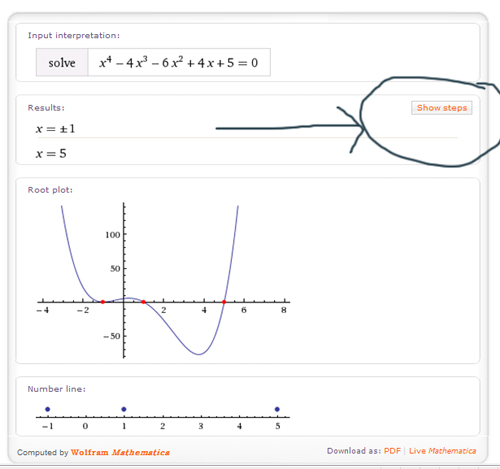﻿ Using Wolfram Alpha to Solve Equations - MathBootCamps

# Using Wolfram Alpha to Solve Equations

If you aren’t familiar with it, Wolfram Alpha is a powerful online tool for calculations and other fun (for example, type in your birthday and see what comes up!). For a student who is trying to understand a new math concept, this tool is great for really taking apart a problem and seeing how it works.

The only downside with wolfram alpha is the input. You have to make sure you state what you want it to do very specifically or it may misinterpret things and give you output that isn’t useful. Today, I will show you how to have it solve equations since this is one of the easier things as far as input goes.

Suppose that we wanted to solve the equation$x^4-4x^3-6x^2+4x+5=0$. In fact, suppose we wanted to find EXACT values (no decimals!). As it is, we would have to use some pretty advanced algebra techniques to get the answer. Heading to wolfram alpha I type in “solve x^4-4x^3-6x^2+4x+5=0”. (I said the input for solving something was easy! The symbol ^ is commonly used for typing exponents)Once you type this in, it is as simple as clicking the equals button or pressing enter on your keyboard! You can see the exact output here. The initial output simply gives the answers and a graph:However, if you click “show steps” that I have circled above, it will show you HOW it got those results making this tool into a powerful solutions manual to help with your studying! Two things to note:

1. Sometimes the steps are unavailable. You will see this in the output link I have given! I have not yet figured out a pattern to this, but sometimes it won’t show the steps. Even when this happens, it still is a great way to at least check answers. (if you are curious how this problem works look at this link for the factorization and set it equal to zero)
2. The steps can be unnatural. You may be thinking of just copying down the steps and handing in the work to your professor or teacher, but if you do this you will be found out pretty easily. A lot of the time the steps are in an order no human would ever use! So, it is a good guide to get started but realize there is likely a nicer way to do things. This is especially true for calculus problems.

So, go play! You can try telling it to “factor”,”solve”, “find” etc. I always try the most direct input and see how it interprets it. Like any tool, you have to understand its limitations and how to work with it well to get any help!## Subscribe to our Newsletter!

We are always posting new free lessons and adding more study guides, calculator guides, and problem packs.

Sign up to get occasional emails (once every couple or three weeks) letting you know what's new!